﻿ Electric potential and charge density around a high voltage wire - XSim

# Electric potential and charge density around a high voltage wire

Update: June 1, 2017
OpenFOAM 4.x

$FOAM_TUTORIALS/electromagnetics/electrostaticFoam/chargedWire ## Summary We will calculate the electric potential Φ and the charge density ρ from the following equations.(Poisson equation)(principle of conservation of charge) where ε0 is the permittivity of free space, j is the current density, and k is the ion mobility. The model geometry is as follows. The region up has a potential of 0 V and a charge gradient of 0. The region hole has a potential of 45000 V and a charge density of 3.57e-05 C/m2. The left, right, and down regions are symmetric boundaries, and the calculation is performed as a two-dimensional problem with a single mesh in the Z direction.Model geometry The meshes are as follows.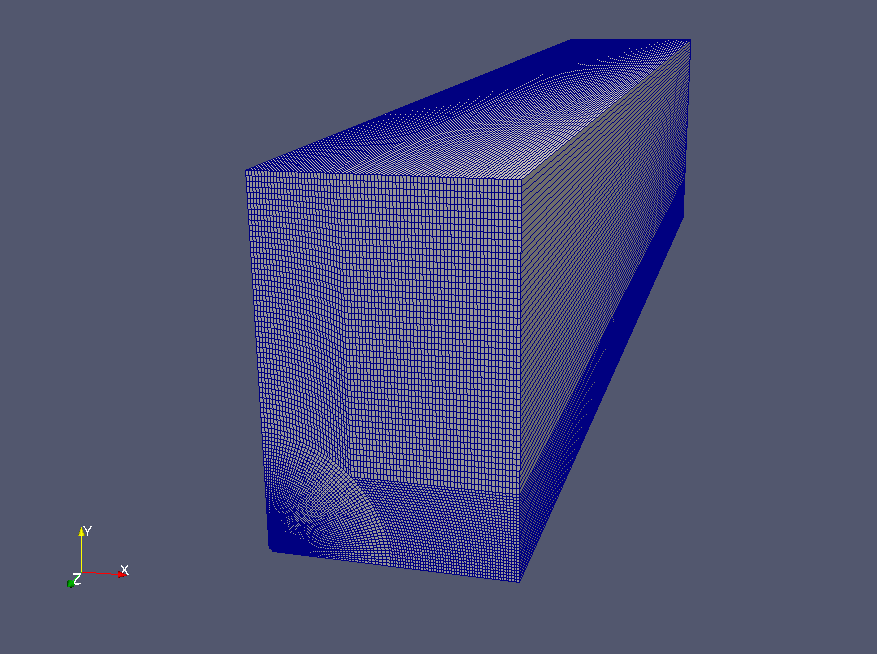Meshes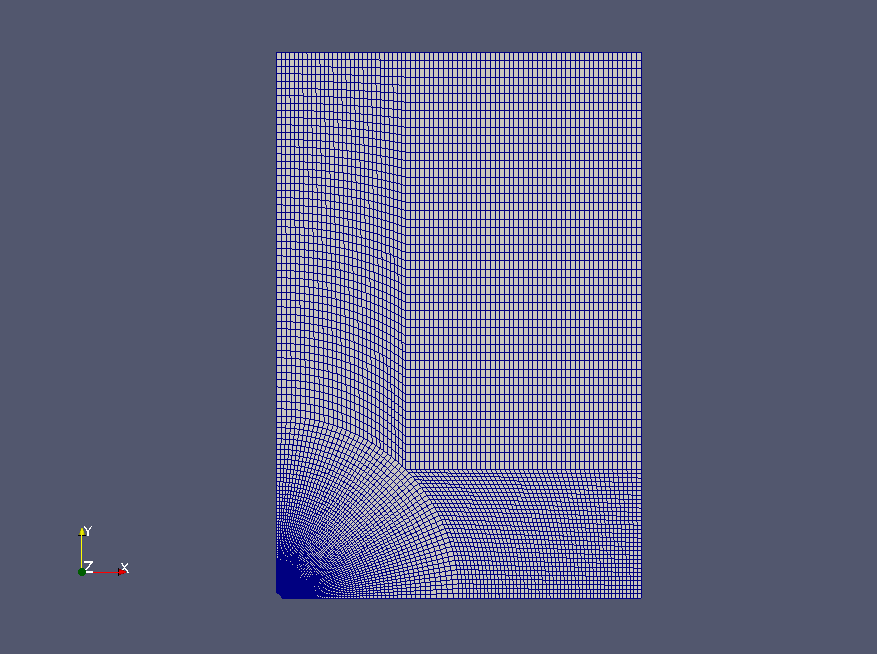Meshes (XY plane) To visualize the calculated potential and charge density, check "phi" and "rho" in the "Properties" tab on ParaView.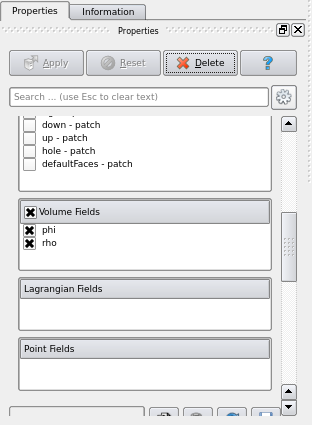Check "phi" and "rho" We can see how the electric potential and charge density decrease around the region "hole".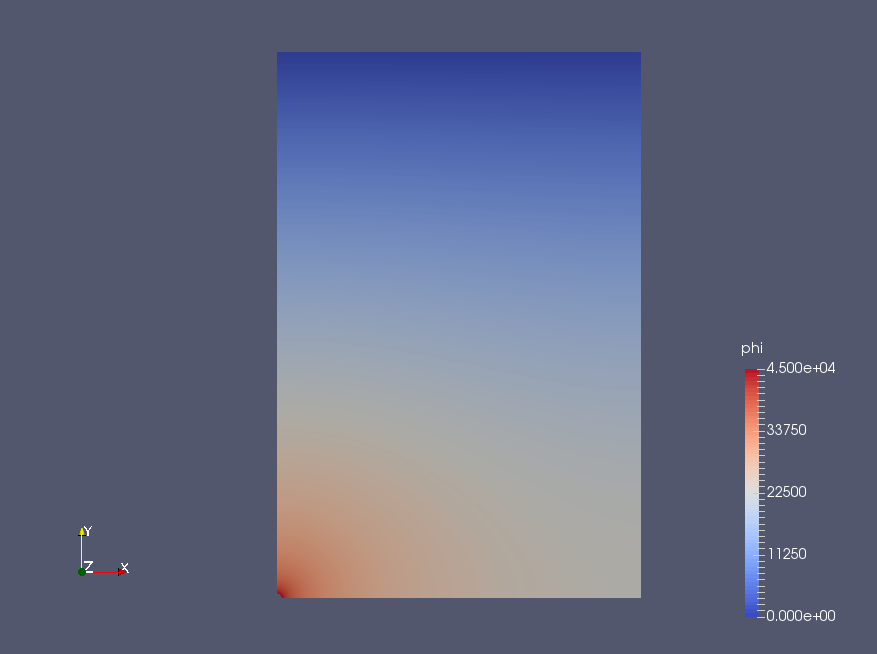Electric potential (phi)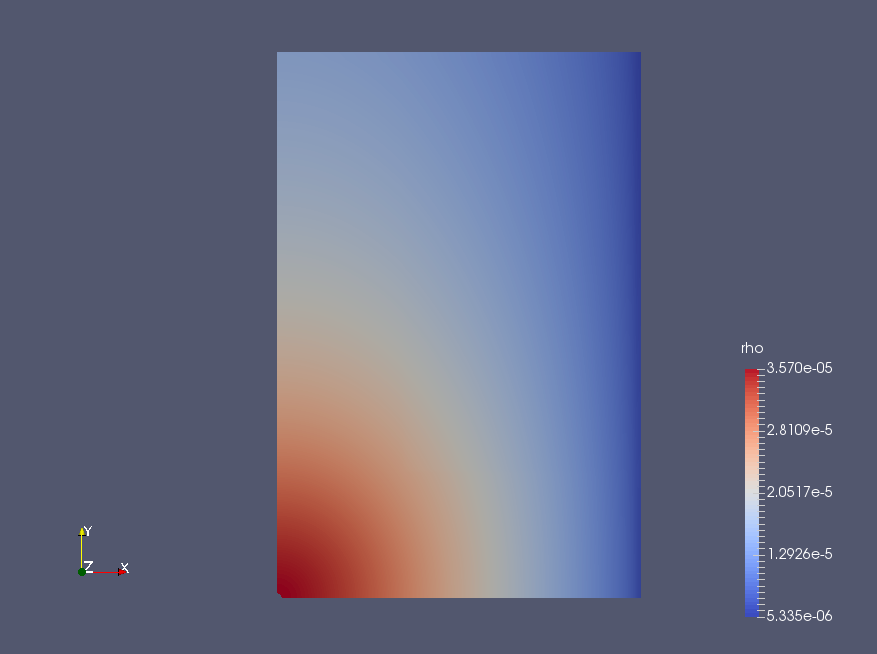Charge density (rho) Also, we can see how the temperature is spread by advection and diffusion. ## Commands cp -r$FOAM_TUTORIALS/electromagnetics/electrostaticFoam/chargedWire chargedWire
cd chargedWire

blockMesh
electrostaticFoam

paraFoam

## Calculation time

--- *Single, Core(TM) i7-2600 CPU @ 3.40GHz 3.40GHz